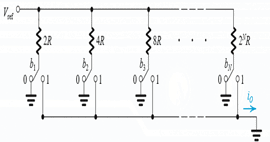Courses

# Test: Signals

## 10 Questions MCQ Test Electronic Devices | Test: Signals

Description
This mock test of Test: Signals for Electrical Engineering (EE) helps you for every Electrical Engineering (EE) entrance exam. This contains 10 Multiple Choice Questions for Electrical Engineering (EE) Test: Signals (mcq) to study with solutions a complete question bank. The solved questions answers in this Test: Signals quiz give you a good mix of easy questions and tough questions. Electrical Engineering (EE) students definitely take this Test: Signals exercise for a better result in the exam. You can find other Test: Signals extra questions, long questions & short questions for Electrical Engineering (EE) on EduRev as well by searching above.
QUESTION: 1

### A cosine wave voltage signal has a 10V RMS value and 60Hz frequency. Also at time, t=0, the value of the voltage signal is equal to its RMS value. Which of the following is the correct mathematical representation of the voltage signal?

Solution:

Only equation 14.14 cos(120πt + π/4) satisfies all the given parameters.

QUESTION: 2

### Which of the following is a characteristic of digital signal?

Solution:

Digital signal is non continuous and has discrete sets of possible value which it can take.

QUESTION: 3

### Consider an N-bits ADC (Analog to Digital Converter) whose analog input varies from 0 to Vmax, then which of the following is not true?

Solution:

None of the statements are true.

QUESTION: 4

In compact disc (CD) audio technology, the signal is sampled at 44kHz. Each sample is represented by 16bits. What is the speed of the system in bits/second?

Solution:

44000 X 16 = 704000 bits/s

QUESTION: 5

An electrical signal can be represented in either Thevenin form or Norton From. However, Thevenin representation is preferred when

Solution:

a is preferred in Thevenin’s case and b in Norton’s case

QUESTION: 6

An electrical signal can be represented in either Thevenin form or Norton From. However, Norton representation is preferred when

Solution:

a is preferred in Thevenin’s case and b in Norton’s case

QUESTION: 7Consider the DAC (digital to Analog Converter) shown below. Where b1, b2….bn can be either 0 or 1. (Q.7 to Q.9)
Q.​ What will be the value of the output current io, if the digital signal is in the form of b1b2b3…bn

Solution:

It is the total current that will flow individual resistances.

QUESTION: 8

Which is the Most Significant Bit and Least Significant Bit in this case?

Solution:

LSB will have maximum resistance (hence least contribution to the total current) and MSB will have least resistance (Hence maximum contribution to the total current).

QUESTION: 9

For vref = 10V, n = 6, and R= 5000 ohm, which of the following is true?

Solution:

According to the figure, the LSB is given by 10 / 50006 mA.

QUESTION: 10

What is the binary representation of 57?

Solution:

The binary equivalent of 57 is 111001